## Introduction

When dealing with an unknown complex system that has a large number of interacting components organized hierarchically, curiosity demands that we ask the following question: are there hidden objects that are not accessible from the external world? The problem of inferring the existence of hidden objects from observations is quite challenging but it has significant applications in many disciplines of science and engineering. Here by “hidden” we mean that no direct observation of or information about the object is available and so it appears to the outside world as a black box. However, due to the interactions between the hidden object and other observable components in the system, it may be possible to utilize “indirect” information to infer the existence of the hidden object and to locate its position with respect to objects that can be observed. The difficulty to develop effective solutions is compounded by the fact that the indirect information on which any method of detecting hidden objects relies can be subtle and sensitive to changes in the system or in the environment. In particular, in realistic situations noise and random disturbances are present. It is conceivable that the “indirect” information can be mixed up with that due to noise or be severely contaminated. The presence of noise thus poses a serious challenge to detecting hidden nodes and some effective “noise-mitigation” method must be developed.

To formulate the problem in a concrete way and to gain insights into the development of a general methodology, we note that the basic principle underlying the detection of hidden objects is that their existence typically leads to “anomalies” in the quantities that can be calculated or deduced from observation. Simultaneously, noise, especially local random disturbances applied at the nodal level, can also lead to large variance in these quantities. This is so because, a hidden node is typically connected to a few nodes in the network that are accessible to the external world and a noise source acting on a particular node in the network may also be regarded as some kind of hidden object. Thus, the key to any detection methodology is to identify and distinguish the effects of hidden nodes on measures for detection from those due to local noise sources.

In this paper, we focus on complex networks and develop a general method to differentiate hidden nodes from local noise sources. This problem is intimately related to the works on reverse engineering of complex networks, where the goal is to uncover the full topology of the network based on measured time series1,2,3,4,5,6,7,8,9,10,11,12,13,14,15,16,17,18,19,20,21,22. Our method is based on the recent work23 on utilizing compressive sensing24,25,26,27,28,29 to detect hidden nodes in the absence of noise sources. To explain our method in a concrete setting, we use the network configuration shown schematically in Fig. 1, where there are 20 nodes, the couplings among the nodes are weighted and the entire network is in a noisy environment, but a number of nodes also receive relatively strong random driving. We assume an oscillator network so that the nodal dynamics are described by nonlinear differential equations and that time series can be measured simultaneously from all nodes in the network except one, labeled as #20, which is a hidden node. The task of ascertaining the presence and locating the position of the hidden node are equivalent to identifying its immediate neighbors, which are nodes #3 and #7 in Fig. 1. Note that, in order to be able to detect the hidden node based on information from its neighboring nodes, the interactions between the hidden node and its neighbors must be directional from the former to the latter or be bidirectional. Otherwise, if the coupling is solely from the neighbors to the hidden node, the dynamics of the neighboring nodes will not be affected by the hidden node and, consequently, time series from the neighboring nodes will contain absolutely no information about the hidden node, which is therefore undetectable. The action of local noise source on a node is naturally directional, i.e., from the source to the node.

Our recent work23 demonstrated that, when the compressive-sensing paradigm is applied to uncovering the network topology15, the predicted linkages associated with nodes #3 and #7 are typically anomalously dense and this piece of information is basically what is needed to identify them as the neighboring nodes of the hidden node. In addition, when different segments of measurement data are used to reconstruct the coupling weights for these two nodes, the reconstructed weights associated with these two nodes exhibit significantly larger variances than those associated with other nodes. However, the predicted linkages associated with the nodes driven by local noise sources can exhibit behaviors similar to those due to the hidden nodes, leading to uncertainty in the detection of the hidden node. To address this critical issue is essential to developing algorithms for real-world applications, which is the aim of this paper. Our main idea is to exploit the principle of differential signal to study the behavior of the predicted link weights as a function of the data used in the reconstruction. Due to the advantage of compressive sensing, the required data amount can be quite small and, hence, even if our method requires systematic increase of the data amount, it will still be reasonably small. We shall argue and demonstrate that, when the various ratios of the predicted weights associated with all pairs of links between the possible neighboring nodes and the hidden node are examined, those associated with the hidden nodes and nodes under strong local noise show characteristically distinct behaviors, rendering unambiguous identification of the neighboring nodes of the hidden node. Any such ratio is essentially a kind of differential signal, because it is defined with respect to a pair of edges.

## Results

We present our results by using coupled oscillator networks. (Results from evolutionary-game dynamical networks are presented in Supporting Information.) Given such a networked system, we use compressive sensing to uncover all the nodal dynamical equations and coupling functions15. This can be done by expanding all the vector fields and functions into series and calculating, from available time series, all the coefficients in the expansion. The expansion base needs to be chosen properly so that the number of non-zero coefficients is small as compared with the total number Nt of unknown coefficients. All Nt coefficients constitute a coefficient vector to be estimated. The amount of data used can be conveniently characterized by Rm, the ratio of the number M of data points used in the reconstruction, to Nt. See Methods.

Our idea to distinguish the effects of hidden node and local noise sources is based on the following observation. Consider two neighboring nodes of the hidden node, labeled as i and j. Because the hidden node is a common neighbor of nodes i and j, the couplings from the hidden node should be approximately proportional to each other, with the proportional constant determined by the ratio of their link weights with the hidden node. When the dynamical equations of nodes i and j are properly normalized, the terms due to the hidden node tend to cancel each other, leaving the normalization constant as a single unknown parameter that can be estimated subsequently. We name this parameter cancellation ratio and denote it by Ωij. As the data amount is increased, Ωij tends to its true value. Practically we then expect to observe systematic changes in the estimated value of the ratio as data used in the compressive-sensing algorithm is increased from some small to relatively large amount. If only local noise sources are present, the ratio should show no systematic change with the data amount. Thus the distinct behaviors of Ωij as the amount of data is increased provides a way to distinguish the hidden node from noise and, at the same time, to ascertain the existence of the hidden node. A mathematical formulation of this general principle can be found in Methods.

We test our method to differentiate hidden nodes and noise using random networks of nonlinear/chaotic oscillators. To be concrete, we choose the nodal dynamics to be that of the Rössler oscillator, one of the classical models in nonlinear dynamics30,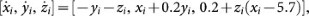which exhibits a chaotic attractor. The size of the network varies from 20 to 100 and the probability of connection between any two nodes is 0.04. The network link weights are equally distributed in [0.1, 0.5] (arbitrary). Background noise of amplitude ξ is applied (independently) to every oscillator in the network, with amplitude varying from 10−4 to 5 × 10−3. The noise amplitude is thus smaller than the average coupling strength of the network. The tolerance parameter ε in the compressive sensing algorithm can be adjusted in accordance with the noise amplitude (see Supporting Information for details). Time series are generated by using the standard Heun's algorithm31 to integrate the stochastic differential equations. To approximate the velocity field, we use third-order polynomial expansions in the compressive-sensing formulation. (In Supporting Information, we present more examples using network systems of varying sizes, different weight distributions and topologies and alternative nodal dynamics.).

### Detecting hidden node from time series

As a concrete example, we consider the network in Fig. 1, where only background noise is present and there are no local noise sources. Linear coupling between any pair of connected nodes is from the z-component to the x-component in the Rössler system. From the available time series (nodes #1–19), we solve the coefficient vector using a standard compressive-sensing algorithm [http://users.ece.gatech.edu/justin/l1magic/]. In particular, for node i, the terms associated with couplings from the z-components of other nodes appear in the ith row of the coupling matrix. As shown in Fig. 2(a), when the data amount is Rm = 0.7, the network's coupling matrix can be predicted. The predicted links and the associated weights are sparse for all nodes except for nodes #3 and #7, the neighbors of the hidden node. While there are small errors in the predicted weights due to background noise, the predicted couplings for the two neighbors of the hidden node, which correspond to the 3rd and the 7th row in the coupling matrix, appear to be from almost all other nodes in the network and some coupling strength is even negative. Such anomalies associated with the predicted coupling patterns of the neighboring nodes of the hidden node cannot be removed by increasing the data amount. Nonetheless, it is precisely these anomalies which hint at the likelihood that these two “abnormal” nodes are connected with a hidden node.

While abnormally high connectivity predicted for a node is likely indication that it belongs to the neighborhood of the hidden node, in complex networks there are hub nodes with abnormally large degrees, especially for scale-free networks32. In order to distinguish a hidden node's neighboring node from some hub node, we use the variance of the predicted coupling constants, which can be calculated from different segments of the available data sets. Due to the intrinsically low-data requirement associated with compressive sensing, the calculation of the variance is feasible because any reasonable time series can be broken into a number of segments and prediction can be made from each data segment. For nodes not in the neighborhood of the hidden node, we expect the variance to be small as the predicted results hardly change when different segments of the time series are used. However, for the neighboring nodes of the hidden node, due to lack of complete information needed to construct the measurement matrix, the variance values can be much larger. Figure 2(b) shows the variance σ2 in the predicted coupling strength for all 19 accessible nodes. We observe that the values of the variance for the neighboring nodes of the hidden node, nodes #3 and #7, are all above the upper dashed line and are in fact significantly larger than those associated with all other nodes that all fall below the lower dashed line. This indicates strongly that they are indeed the neighboring nodes of the hidden node. The gap between the two dashed lines can be taken as a quantitative measure of the detectability of the hidden node. The larger the gap, the more reliable it is to distinguish the neighbors of the hidden node from the nodes that not in the neighborhood. The results in Fig. 2 thus indicate that the locations of the hidden node(s) in the network can be reliably inferred even in the presence of weak background noise. The size of the gap, or the hidden-node detectability depends on the system details. In Supporting Information, we present results of a systematic analysis of the detectability measure, where we find that the variance due to the hidden nodes is mainly determined by the strength of their coupling with the accessible nodes in the network. We also find that system size and network topology have little effect on the hidden-node detectability. It is worth emphasizing that the detectability relies also on successful reconstruction of all nodes that are not in the neighborhood of the hidden nodes, which determine the lower dashed line in Fig. 2.

To quantify the reliability of the reconstruction results, we investigate how the prediction errors in the link weights of all accessible nodes, except the predicted neighbors of the hidden node, change with the data amount. For an existent link, we use the normalized absolute error Enz, the error in the estimated weight with respect to the true one, normalized by the value of the true link weight. Figure 3 shows the results for N = 100. The link weights are uniformly distributed in the interval [0.1, 0.5] and the background noise amplitude is ξ = 10−3. The tolerance parameter in the compressive-sensing algorithm is set to be ε = 0.5, which is optimal for this noise amplitude. (In Supporting Information we provide details of determining the optimal tolerance parameter for different values of the background noise amplitude.) We see that for Rm > 0.4, Enz decreases to the small value of about 0.01, which is determined by background noise level. As Rm is increased further, the error is bounded by a small value determined by the noise amplitude, indicating that the reconstruction is robust. Although the value of Enz does not decrease further toward zero due to noise, the prediction results are reliable in the sense that the predicted weights and the real values agree with each other, as shown in the inset of Fig. 3, a comparison of the actual and the predicted weights for all existent links. All the predicted results are in the vicinities of the corresponding actual values, as indicated by a heavy concentration of the dots along the diagonal line. The central region in the dot distribution has brighter color than the marginal regions, confirming that vast majority of the predicted results are accurate. In Supporting Information, we further show that robust reconstruction can be achieved regardless of the network size, connection topology and weight distributions, insofar as sufficient data are available.

The error measure Enz to characterize the accuracy of the reconstruction is similar to z-scores, or the standard score in statistics, with the minor difference being that z-scores use the standard derivatives of the distribution to normalize the raw scores, while we use the exact values in our model examples. In realistic applications the exact values are usually not available, so it is necessary to use the z-score measure.

We emphasize that there are two types of “dense” connections: one from reconstruction and another intrinsic to the network. In particular, in the two-dimensional representation of the reconstruction results [e.g., Fig. 2(A)], the neighboring nodes of the hidden node typically appear densely linked to many other nodes in the network. These can be a result of lack of incomplete information (i.e., time series) due to the hidden node (in this case, there is indeed a hidden node), or the intrinsic dense connection pattern associated with, for example, a hub node in a scale-free network. Our idea of examining the variances of the reconstructed connections from independent data segments is for distinguishing these two possibilities. As we have demonstrated, extensive computations indicate that a combination of the dense connection and large variance can ascertain the existence of hidden node reliably.

### Differentiating hidden node from local noise sources

When strong noise sources are present at certain nodes, the predicted coupling patterns of the neighboring nodes of these nodes will show anomalies. (Here by “strong” we mean that the amplitudes of the random disturbances are order-of-magnitude larger than that of background noise.) We now demonstrate that our proposed method based on the cancellation ratio is effective at distinguishing hidden nodes from local noise sources, insofar as the hidden node has at least two neighboring nodes not subject to such disturbances. To be concrete, we choose a network of N = 61 coupled chaotic Rössler oscillators, which has 60 accessible nodes and one hidden node (#61) that is coupled to two neighbors: nodes #14 and #20, as shown schematically in Fig. 4. Assume a strong noise source is present at node #54. We find that the reconstructed weights match their true values to high accuracy. We also find that the reconstructed coefficients including the ratio Ωij are all constant and invariant with respect to different data segments, a strong signal that the pair of nodes are the neighboring nodes of the same hidden node, thereby confirming its existence.

When there are at least two accessible nodes in the neighborhood of the hidden node which are not subject to strong noisy disturbance, such as nodes #14 and #20, as the data amount Rm is increased towards 100%, the cancellation ratio should also increase and approach unity. This behavior is shown by the open circles in Fig. 5(a). However, when a node is driven by a local noise source, regardless of whether it is in the neighborhood of the hidden node, the cancellation ratio calculated from this node and any other accessible node in the network will show a characteristically different behavior. Consider, for example, nodes #14 and #54. The reconstructed connection patterns of these two nodes both show anomalies, as they appear to be coupled with all other nodes in the network. In contrast to the case where the pair of nodes are influenced by the hidden node only, here the cancellation ratio does not show any appreciable increase as the data amount is increased, as shown by the crosses in Fig. 5(a). In addition, the average variance values of the predicted coefficient vectors of the two nodes exhibit characteristically different behaviors, depending on whether any one node in the pair is driven by strong noise or not. In particular, for the node pair #14 and #20, since neither is under strong noise, the average variance will decrease toward zero as Rm approaches unity, as shown in Fig. 5(b) (open circles). In contrast, for the node pair #14 and #54, the average variance will increase with Rm, as shown in Fig. 5(b) (crosses). This is because, when one node is under strong random driving, the input to the compressive-sensing algorithm will be noisy and its performance will deteriorate. However, compressive sensing can perform reliably when the input data are “clean,” even when they are sparse. Increasing the data amount beyond a threshold is not necessarily helpful, but longer and noisy data sets can degrade significantly the performance. The results in Figs. 5(a,b) thus demonstrate that the cancellation ratio between a pair of nodes, in combination with the average variance of the predicted coefficient vectors associated with the two nodes, can effectively distinguish a hidden node from a local noise source. If there are more than one hidden node or there is a cluster of hidden nodes, the procedure to estimate the cancellation factors is similar but requires additional information about the neighboring nodes of the hidden nodes. Our cancellation-factor based method can be extended to network systems with nodal dynamics not of the continuous-time type, such as evolutionary-game dynamics. See Supporting Information for details.

## Discussion

Our program to differentiate a hidden node from local noise sources and then to infer its existence can be summarized into the following steps:

• Collect time series of dynamical variables from accessible nodes;

• Hypothesize suitable expansion bases for nodal dynamics and coupling functions, taking advantage of physical understanding of the underlying networked dynamical system;

• Construct the measurement matrix and derivative vector from time series and solve the expansion-coefficient vector using compressive sensing;

• Identify all nodes with abnormally dense connections and calculate the corresponding variances using independent segments of the available time series to eliminate the hub nodes in the network (for those nodes the variances will be much smaller than those of the neighboring nodes of the hidden node or nodes under strong local noise);

• For all the remaining nodes with abnormally dense connections, calculate the cancellation ratio for all possible node pairs and also the average variance of the predicted coefficient vectors using non-overlapping time-series segments for a series of systematically increasing values of the data amount Rm;

• Identify the neighboring nodes of the hidden node as those with cancellation ratios approaching unity and the average variance tending to zero as Rm is increased. For those pairs with cancellation ratio not increasing and/or the average variance not decreasing with Rm, one node in the pair is under the driving of a local noise source.

Detecting hidden nodes in complex networks with a priori unknown nodal dynamics, topology and coupling weights has vast application potential, such as in social and biological networks. Inferring the existence of hidden node in the presence of local random perturbations is an extremely challenging problem. Our efforts represent a step forward in this area of research, where much further work is needed.

## Methods

### Compressive-sensing based method to uncover network dynamics and topology

We consider the typical setting of a complex network of N coupled oscillators in a noisy environment. The dynamics of each individual node, when it is isolated from other nodes, can be described as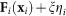, where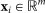is the vector of state variables and ηi are an m-dimensional vector whose entries are independent Gaussian random variables of zero mean and unit variance and ξ denotes the noise amplitude. A weighted network can be described by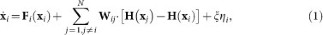where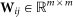is the coupling matrix between node i and node j and H is the coupling function. Defining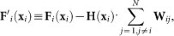we have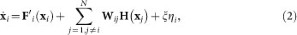i.e., we have grouped all terms directly associated with node i into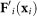. We can then expand F′(xi) into the following form: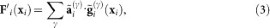where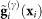are a set of orthogonal and complete base functions chosen such that the coefficients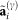are sparse. While the coupling function H(xi) can be expanded in a similar manner, for simplicity we assume that they are linear: H(xi) = xi. We then have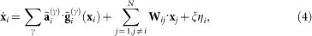where all the coefficients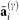and weights Wij need to be determined from time series xi. In particular, the coefficient vector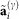determines the nodal dynamics and the weighted matrices Wij's give the full topology and coupling strength of the entire network.

Suppose we have simultaneous measurements of all state variables xi(t) and xi(t + δt) at M different uniform instants of time at interval Δt apart, where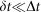so that the derivative vector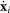can be estimated at each time instant. Equation (4) for all M time instants can then be written in a matrix form with the following measurement matrix: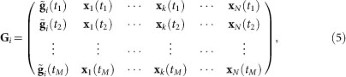where the index k in xk(t) runs from 1 to N, ki and each row of the matrix is determined by the available time series at one instant of time. The derivatives at different time can be written in a vector form as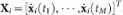and the coefficients from the functional expansion and the weights associated with all links in the network can be combined concisely into a vector ai as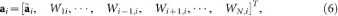where [·]T denotes the transpose. For properly chosen expansion base and a general complex network whose connections are typically sparse, the vector ai to be determined is sparse as well. Finally, Eq. (4) can be written asIn the absence of noise or if the noise amplitude is negligibly small, Eq. (7) represents a linear equation but the dimension of the unknown coefficient vector ai can be much larger than that of Xi and the measurement matrix will have many more columns than rows. In order to fully recover the network of N nodes with each node having m components, it is necessary to solve N × m such equations.

### Recovering signal from noisy measurement with compressive sensing algorithm

The system of linear equations in Eq. (7 is ill defined. However, since ai is sparse, insofar as its number of non-zero coefficients is smaller than the dimension of Xi, the vector ai can be uniquely and efficiently determined by the compressive-sensing algorithm24,25,26,27,28,29. In particular, in the equation X = G · a + ξ, reliable recovery of the P-dimension sparse vector a is achievable, according to25, where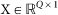and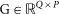but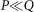. A sufficiently sparse vector a can be reconstructed by solving the following l1 regularization problem: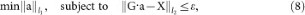where the l1 norm for a vector x is defined as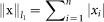, its l2 norm is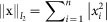, ε is the threshold value determined by the noise amplitude. The reconstructed vectorlies within the range: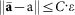, where C is a constant.

### Detection of hidden node

To motivate our consideration, we note that, a meaningful solution of Eq. (7) based on compressive sensing requires that the derivative vector Xi and the measurement matrix Gi be entirely known which, in turn, requires time series from all nodes. In this case, we say that information available for reconstruction of the complex networked system is complete. In the presence of a hidden node, for its immediate neighbors, the available information will not be complete in the sense that some entries of the vector Xi and the matrix Gi become now unknown. Let h denote the hidden node. For any neighboring node of h, the vector Xi and the matrix Gi in Eq. (7) now contain unknown entries at the locations specified by the index h. For any other node not in the immediate neighborhood of h, Eq. (7) is unaffected. When compressive-sensing algorithm is used to solve Eq. (7), there will then be large errors in the solution of the coefficient vector ai associated the neighboring nodes of h, regardless of the amount of data used. In general, the so-obtained coefficient vector ai will not appear sparse. Instead, most of its entries will not be zero, a manifestation of which is that the node would appear to have links with almost every other node in the network. In contrast, for nodes not in the neighborhood of h, the corresponding errors will be small and can be reduced by increasing the data amount and the corresponding coefficient vector will be sparse. It is this observation which makes identification of the neighboring nodes of the hidden node possible in the noiseless or weak-noise situations23.

To appreciate the need and the importance to distinguish the effects of hidden node from these of noise, we can separate the term associated with h in Eq. (4) from those with other accessible nodes in the network. Letting l denote a node in the immediate neighborhood of the hidden node h, we have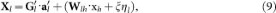where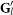is the new measurement matrix that can be constructed from all available time series. While background noise may be weak, the term Wlh · xh can in general be large in the sense that it is comparable in magnitude with other similar terms in Eq. (4). Thus, when the network is under strong noise, especially for those nodes that are connected to the neighboring nodes of the hidden node, the effects of hidden node on the solution can be entangled with those due to noise. In addition, if the coupling strength from the hidden node is weak, it would be harder to identify the neighboring nodes. For example, hidden node in a network with Gaussian weight distribution will be harder to detect, due to the finite probability of the occurrence of near zero weights. When the coupling strength is comparable or smaller than the background noise amplitude, the corresponding link cannot be detected. See Supporting Information for details.

### Method to distinguish hidden nodes from local noise sources - a mathematical formulation

For simplicity, we assume that all coupled oscillators share the same coupling scheme and that each oscillator is coupled to any of its neighbors through one component of the state vector only. Thus, each row in the coupling matrix Wih associated with a link between node i and h has only one non-zero element. Let p denote the component of the hidden node coupled to the first component of node i, the dynamical equation of which can then be written as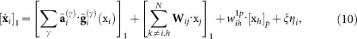where [xh]p denotes the time series of the pth component of the hidden node, which is unavailable andis the coupling strength between the hidden node and node i. The dynamical equation of the first component of neighboring node j of the hidden node has a similar form. Letting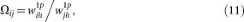be the cancellation ratio, multiplying Ωij to the equation of node j and subtracting from it the equation for node i, we obtain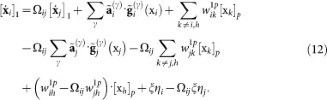We see that terms associate with [xh]p vanish and all deterministic terms on the left-hand side of Eq. (12) are known, which can then be solved by the compressive-sensing method. From the coefficient vector so estimated, we can identify the coupling of nodes i and j to other nodes, except for the coupling between themselves since such terms have been absorbed into the nodal dynamics and the couplings to their common neighborhood are degenerate in Eq. (12) and cannot be separated from each other. Effectively, we have combined the two nodes together by introducing the cancellation ratio Ωij.

To give a concrete example, we consider the situation where each oscillator has three independent dynamical variables, named as x, y and z. For the nodal and coupling dynamics we choose polynomial expansions of order up to n. The x component of the nodal dynamics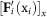for node i is: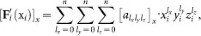and the coupling from other node k to the x component can be written as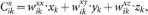where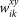denotes the coupling weight from the y component of node k to the x component of node i and so on. The nodal dynamical terms in the matrix Gi are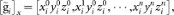and the corresponding coefficients are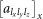. The vector of coupling weights is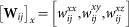. Equation (12) becomes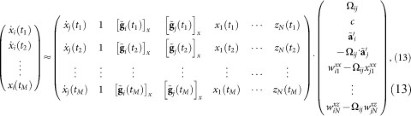where c is the sum of constant terms from the dynamical equations of nodes i and j and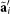is the coefficient vector to be estimated which excludes all the constants. Using compressive sensing to solve Eq. (13), we can recover the cancellation ratio Ωij and the equations of node i. When Ωij is known we can then recover the dynamics of node j from the coefficient vector.

In Supporting Information we provide an analysis and discussion about the possible extension of our method to systems of characteristically different nodal dynamics and/or with multiple hidden nodes. In particular, we show that the method can be readily adopted to network systems whose nodal dynamics are not described by continuous-time differential equations but by discrete-time processes such as evolutionary-game dynamics. In such a case, the derivatives used for continuous-time systems can be replaced by the agent payoffs. The cancellation factors can then be calculated from data to differentiate the hidden nodes from local noise sources. We also show that, under certain conditions with respect to the coupling patterns between the hidden nodes and their neighboring nodes, the cancellation factors can be estimated even when there are multiple, entangled hidden nodes in the network.